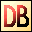DelphiBasics
 PtInRectFunction Tests to see if a point lies within a rectangle Types unit
 function PtInRect(const TheRectangle TRect; const ThePoint TPoint):Boolean;
Description
The PtInRect function returns true if ThePoint lies within TheRectangle.

Note that the rectangle inside is defined as:

(left, top, right-1, bottom-1)
Related commands
 Bounds Create a TRect value from top left and size values Point Generates a TPoint value from X and Y values PointsEqual Compares two TPoint values for equality Rect Create a TRect value from 2 points or 4 coordinates TPoint Holds X and Y integer values TRect Holds rectangle coordinate valuesDownload this web site as a Windows program.

 Example code : Determine inside and outside points of a rectangle var   myRect : TRect; begin   // Create a rectangle   // Note : The rectangle inisde starts at top left, but ends   //        1 pixel inside bottom right.   myRect := Rect(20, 30, 100, 200);   // Check to see if (20,30) is inside the rectangle   if PtInRect(myRect, Point(20,30))   then ShowMessage(' 20, 30 is  inside the rectangle')   else ShowMessage(' 20, 30 is outside the rectangle');   // Check to see if (99,199) is inside the rectangle   if PtInRect(myRect, Point(99,199))   then ShowMessage(' 99,199 is  inside the rectangle')   else ShowMessage(' 99,199 is outside the rectangle');   // Check to see if (100,200) is inside the rectangle   if PtInRect(myRect, Point(100,200))   then ShowMessage('100,200 is  inside the rectangle')   else ShowMessage('100,200 is outside the rectangle'); end; Show full unit code 20, 30 is inside the rectangle    99,199 is inside the rectangle   100,200 is outside the rectangle